# CD Get Data from Model VI

LabVIEW 2018 Control Design and Simulation Module Help

Edition Date: March 2018

Part Number: 371894J-01

»View Product InfoDownload Help (Windows Only)

Owning Palette: Model Information VIs

Requires: Control Design and Simulation Module

Obtains data that describes the dynamics of the given system model. Wire data to the State-Space Model input to determine the polymorphic instance to use or manually select the instance.

Use the pull-down menu to select an instance of this VI.

 Select an instance CD Get Data from Model (State-Space)CD Get Data from Model (Transfer Function)CD Get Data from Model (Zero-Pole-Gain)

## CD Get Data from Model (State-Space)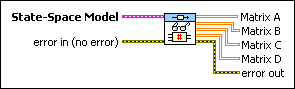State-Space Model contains a mathematical representation of and information about a linear time-invariant (LTI) system for which this VI returns data.error in describes error conditions that occur before this node runs. This input provides standard error in functionality.Matrix A returns the system matrix that describes the dynamics of the states of the system.Matrix B returns the input matrix of the system that relates the inputs to the states.Matrix C returns the output matrix that relates the outputs to the states.Matrix D returns the transmission matrix that relates the inputs to the outputs.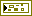error out contains error information. This output provides standard error out functionality.

## CD Get Data from Model (Transfer Function)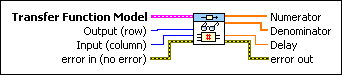Transfer Function Model contains a mathematical representation of and information about a linear time-invariant system for which this VI returns data.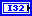Output (row) specifies the output, or row, number of the transfer function in which this VI retrieves the data. This number is necessary when the model represents a MIMO system. The default value is zero.Input (column) specifies the index number of the input column of the transfer function in which this VI retrieves the data. This number is necessary when the model represents a MIMO system. The index is zero-based. The default is zero.error in describes error conditions that occur before this node runs. This input provides standard error in functionality.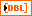Numerator returns the numerator of the transfer function as Output (row) and Input (column) specify.Denominator returns the denominator of the transfer function as Output (row) and Input (column) specify.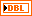Delay returns the transport time delay between the Output (row) and Input (column) pair.error out contains error information. This output provides standard error out functionality.

## CD Get Data from Model (Zero-Pole-Gain)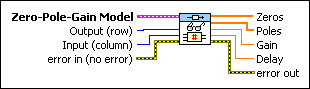Zero-Pole-Gain Model contains a mathematical representation of and information about a linear time-invariant system for which this VI returns data.Output (row) specifies the output, or row, number of the transfer function (in zero-pole-gain form) in which this VI returns data. This number is necessary when the model represents a MIMO system. The index is zero-based. The default value is zero.Input (column) specifies the index number of the input column of the transfer function (in zero-pole-gain form) in which this VI returns data. This number is necessary when the model represents a MIMO system. The index is zero-based. The default is zero.error in describes error conditions that occur before this node runs. This input provides standard error in functionality.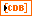Zeros returns the zeros of the SISO system as Output (row) and Input (column) specify.Poles returns the poles of the SISO system as Output (row) and Input (column) specify.Gain returns the scalar gain of the SISO system as Output (row) and Input (column) specify.Delay returns the transport time delay between the Output (row) and Input (column) pair.error out contains error information. This output provides standard error out functionality.